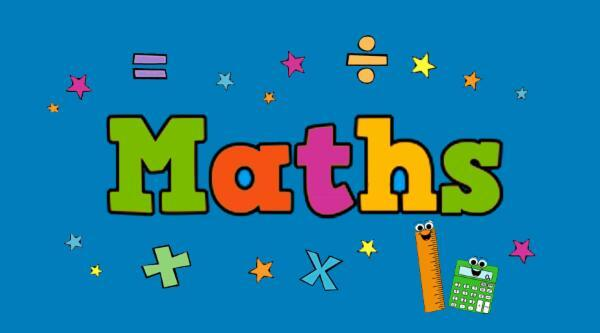👋🏼 Var med och förbättra Skolbanken med oss på Unikum. Svara på formuläret här

# Maths BLIC 1

Skapad 2021-09-13 22:00 i Kvarngärdesskolan Uppsala
Grundskola 1 – 3 MatematikYou will work with several different problem solving solutions, work and consolidate mathematical concepts, methods and forms of expression. You will also be given many opportunities to reason math in groups and with your peers.

## What we will be working on:

#### Number perception and use of numbers

• Numbers 0-12
• Write the numbers correctly
• Number line
• The concept more than, less than, equal to/just as many
• Characters =, <, >
• Splitting numbers
• Worded math problems
• Money 0-20
• Subtraction, with two or three numbers and showing it on the number line
• The relationship between addition and subtraction

#### Algebra

• The meaning of the equal sign
• Missing numbers (2+_ = 5)
• Continue a geometric pattern

#### Geometry

• Tangram
• Draw simple figures from grid systems

#### Probability and statistics

• Bar chart - fill in, compare, talk about results and compose a conclusion

#### Problem solving

• Money
• Math-word problems
• Formulate mathematical expressions based on math-word problems

## How?

• We will work with the math book "Favorit Matematik 1A".
• There will be exercises and reviews together in class and sometimes in smaller groups.
• We will write and count numbers.
• We will work and try different solutions for tasks and problems.
• We will discuss.
• We will play various math games.

## Assessment:

• Participation and work during lessons.
• A summative test is done at the end of each chapter.

What we work with during the autumn term is the basis for achieving the knowledge requirements in year 3, shown below.

### Kopplingar till läroplanen

• Syfte
• formulera och lösa problem med hjälp av matematik samt värdera valda strategier och metoder,
Ma
• använda och analysera matematiska begrepp och samband mellan begrepp,
Ma
• välja och använda lämpliga matematiska metoder för att göra beräkningar och lösa rutinuppgifter,
Ma
• föra och följa matematiska resonemang, och
Ma
• använda matematikens uttrycksformer för att samtala om, argumentera och redogöra för frågeställningar, beräkningar och slutsatser.
Ma
• Centralt innehåll
• Naturliga tal och deras egenskaper samt hur talen kan delas upp och hur de kan användas för att ange antal och ordning
Ma  1-3
• Matematiska likheter och likhetstecknets betydelse.
Ma  1-3
• Hur enkla mönster i talföljder och enkla geometriska mönster kan konstrueras, beskrivas och uttryckas
Ma  1-3
• Grundläggande geometriska objekt, däribland punkter, linjer, sträckor, fyrhörningar, trianglar, cirklar, klot, koner, cylindrar och rätblock samt deras inbördes relationer. Grundläggande geometriska egenskaper hos dessa objekt.
Ma  1-3
• Enkla tabeller och diagram och hur de kan användas för att sortera data och beskriva resultat från enkla undersökningar, såväl med som utan digitala verktyg.
Ma  1-3
• Olika proportionella samband, däribland dubbelt och hälften.
Ma  1-3
• Matematisk formulering av frågeställningar utifrån enkla vardagliga situationer.
Ma  1-3